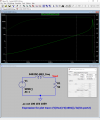Inductance q simulation in ltspice

Kevil

Joined Jun 28, 2020
95
I am not sure if I simulated correctly the Q = 84 for 1.575 GHz in LTspice for Coilcraft inductance 0402DC 8.2 nF Model libraries for LTspice are here (lower right corner).

The next question is if it is possible to replace the trace expression to plot by function i.e. to define the expression in the schematic and use the function result as the expression to plot. I mean something like:

Q = (V(Vout)-V(n001))/Ix(U1ort2) and as "expression to plot" to use Q only.Attachments

• 657 bytes Views: 4

ericgibbs

Joined Jan 29, 2010
14,693
hi Kevil,
We need the inductor model.
E

eetech00

Joined Jun 8, 2013
2,808
I am not sure if I simulated correctly the Q = 84 for 1.575 GHz in LTspice for Coilcraft inductance 0402DC 8.2 nF Model libraries for LTspice are here (lower right corner).

The next question is if it is possible to replace the trace expression to plot by function i.e. to define the expression in the schematic and use the function result as the expression to plot. I mean something like:

Q = (V(Vout)-V(n001))/Ix(U1ort2) and as "expression to plot" to use Q only.

View attachment 254243
I think the coilcraft models have an issue with the coding for LTspice.
The laplace statement must contain static params only (besides "s" of course).
In addition, caret (^) character is an XOR function, so they would need to be replaced with ** to specify "to the power of".

Kevil

Joined Jun 28, 2020
95
hi Kevil,
We need the inductor model.
E

I have attached the 0402DC.lib

Attachments

• 86.2 KB Views: 0
• 382 bytes Views: 1
Last edited:

eetech00

Joined Jun 8, 2013
2,808
•ericgibbs